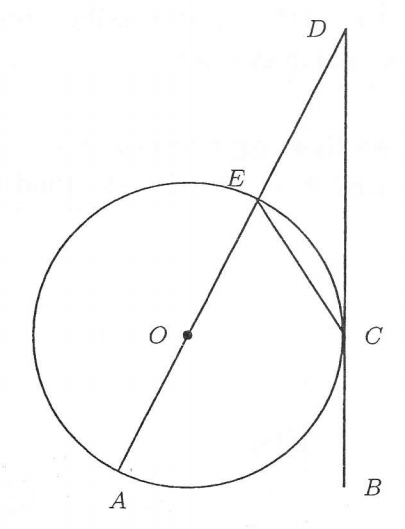# Angle with tangent

Geometry Level 3In the figure above, the line $BD$ is tangent to the circle at $C$. The line $AD$ passes through the centre $O$ of the circle and intersects the circle at $E$.

It is given that $\angle CDE=34^{\circ}$ and $\angle DCE=x^{\circ}$.

Find the value of $x$.

×